## Generation of high order geometry representations in Octree meshes

Note that a Preprint of this article also exists, first published August 20, 2015.

## Introduction

High-order approximations are attractive for numerical simulations on modern computing systems due to their fast error convergence. They can solve complex problems accurately with few degrees of freedom and therefore, low memory consumption. This is an important property, as memory is an expensive resource in nowadays computing systems.

The discontinuous Galerkin finite element method (DGFEM) is a relatively recent numerical scheme, gaining attraction in a wide range of application domains. An introduction and overview is offered by Hesthaven & Warburton (2007). Besides the possibility to use high-order approximations, the local basis definition in DGFEM nicely fits the demands of massively parallel and distributed computing systems. Thus, a high-order DGFEM discretization is a good candidate to solve large scale problems.

However, one drawback of high-order methods is the need for appropriate descriptions of geometrical setups in the simulation with the same approximation accuracy as the numerical scheme. We present a method to obtain such a description for inhomogeneous material distributions. Specifically, we consider non-smooth distributions, with a clear interface between distinct material properties. Such variations in material parameters appear for example in the field of electrodynamics, and we will use a simple scattering by a cylindrical object to highlight the application of the generated material in a DGFEM solver. Electrodynamics is governed by Maxwell’s equations and with an isotropic, linear material they read: $\nabla \cdot \left(\epsilon \mathbf{E}\right)={\rho }^{e}$ $\nabla \cdot \mathbf{B}=0$ $\frac{\partial \mathbf{B}}{\partial t}+\nabla ×\mathbf{E}=0$ $\frac{\partial \epsilon \mathbf{E}}{\partial t}-\nabla ×\left(\mathbf{B}/\mu \right)=-\mathbf{j}.$

Gauss’s law (1) states a direct relation between the divergence of the electrical field E and the electrical charge density ρe. Similarly, the magnetic field B has to be divergence free as there are no magnetic charges, which is expressed in (2). The two fields evolve in time according to Faraday’s (3) and Ampère’s law (4). In these equations, the environment is described by permittivity ε and permeability μ of present materials. Our goal is the conversion of surface descriptions that separate regions of different materials into functions of space ε(x, y, z) and μ(x, y, z). Though, we will only consider Maxwell’s equations in this work, the method is generic and can be used for any partial differential equation with spatially varying material parameters.

We consider here a DGFEM with polynomial basis functions, and therefore, generate polynomial representations locally in each element. Geometry identification is tightly related to mesh generation. Therefore, we integrated this method into our meshing tool Seeder (Klimach et al., 2015), which is freely available under an open-source licence. Seeder creates meshes with cubical elements based on an Octree refinement towards boundaries. It uses STL (White, 2013) files to describe boundary surfaces of arbitrary complexity. With the method, we present here, the cubical elements can now be equipped with additional information to provide the spatial distribution of materials within the cubical elements.

The fundamental idea to obtain high-order polynomial representations is the use of a simple first-order voxelization within the coarse elements of the mesh for the DGFEM solver. This volume information can then be translated into polynomials by a suitable transformation method. A major feature of the first-order method is its robustness that allows the treatment of complex setups. A drawback is its low accuracy and need for a large number of voxels. However, this is alleviated by using the Octree strategy of recursive refinement. It thereby gets feasible to generate accurate polynomials of high-order for arbitrary surfaces.

## Related Work

Our approach is most closely related to embedded boundaries, as used in spectral discretizations. Examples of such approaches are the Spectral Smoothed Boundary Method (Bueno-Orovio, Pérez-García & Fenton, 2006) and the Fourier Spectral Embedded Boundaries (Sabetghadam, Sharafatmandjoor & Norouzi, 2009). These methods rely on a representation of the irregular domain by a function. This function, also referred to as phase-field, is usually constructed with the help of a global rectangular Cartesian grid. We extend this concept with an Octree mesh, where we apply this method in each of the elements of the mesh. The constructed geometry representation is then defined locally within each mesh cell. This matches the function space of the DGFEM and can be directly used by DGFEM solvers. Within the elements, we also make use of the Octree bisection algorithm to achieve a fast voxelization of surfaces. In comparison to a global rectangular domain in spectral methods, the composition of multiple elements in a mesh allows for a greater flexibility in the definition of the embedding domain. By fitting the embedding domain to the actual computational domain of interest, the computational effort can be minimized in this approach.

Other methods, where irregular meshes are deployed with an internal geometry representation are typically referred to as Immersed Boundary Methods. Introduced by Peskin (2002) for elastic walls in incompressible flows, this approach has been improved and extended since by various authors. An overview of these methods is for example provided by Mittal & Iaccarino (2005). Similarly to the strategy we follow here, these methods make use of meshes for the computational domain. However, they rely on surface representations to describe objects within the meshes and directly enforce boundary conditions on these. They are popular for flows with moving geometries but do not provide a direct method to describe varying materials, like the change in permeability and permittivity in electrodynamics. In contrast to the embedded boundaries in spectral methods, the immersed boundaries are usually employed in lower order schemes.

Our goal is the generation of material representations for high-order DGFEM solvers. As such, we need a mesh like in the immersed boundary methods, but a high-order functional representation of the geometry as in the embedded boundary methods. This method enables the exploitation of the fast convergence of spectral approximations but still allows for complex computational domains.

The traditional approach to boundaries in unstructured, irregular meshes is the fitting of the mesh to the geometry. Here, a high-order can be obtained by deforming the elements with curved surfaces. Hindenlang, Bolemann & Munz (2015) offers a method in this direction specifically designed for discontinuous Galerkin discretizations. However, the identification of such curved boundaries is much more complex and usually not used for internal interfaces like material changes, as both sides of the interface need to be considered. Such deformed, unstructured elements are also subject to varying mesh quality and prone to issues with geometrical constraints. As we will show, the embedding description of materials provides a viable and robust approach to the body fitting of meshes. It comes at the cost of volumetric information that needs to be stored but avoids the need for expensive transformations during the simulation.

## The Seeder Mesh Generator

Seeder (Harlacher et al., 2012) is an Octree mesh generator. It produces voxelizations of complex geometries defined by surface triangulations in the form of STL files. Some geometric primitives, like spheres and cylinders, are also available, and we will make use of them in the examples considered here. By voxelization, we refer to the process of subdividing a given volume into smaller cubical elements (voxels) in three-dimensional space. With the Octree approach, these cubical elements are successively split into 8 smaller cubes, where needed. An example for the voxelization of a sphere is shown in Fig. 1. The yellow surface indicates the sphere and the red color indicates the voxels completely inside the sphere. Note how the voxels build a staircase that approximates the smooth surface. Also, the Octree refinement is visible in Fig. 1. Our concept of voxels does not imply equally sized voxels. Instead, as outlined by the white lines, different voxel sizes arise from the bisection rule of the Octree approach. Thus, we only need to create a large number of small voxels close to the smooth surface while covering the rest of the volume with just a few large ones. The mesh format generated by Seeder exploits the topology information from the Octree and is designed for the parallel processing on distributed, parallel systems. Seeder is freely available online (Klimach et al., 2015) under a permissive BSD license and has been successfully compiled and run on many computing architectures. In the following section, the general voxelization method is briefly outlined. Afterward, we explain the extensions to enable high-order material definitions within the mesh elements.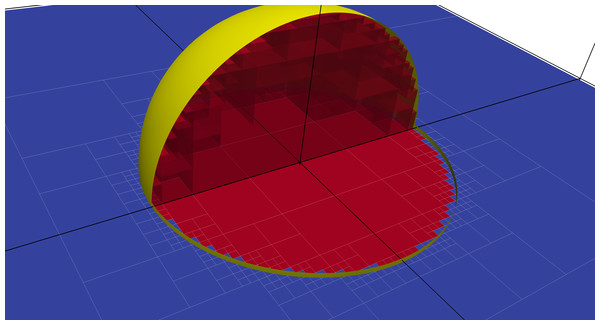Figure 1: Illustration of the voxelization of a sphere within coarse mesh elements. The sphere is indicated by the yellow surface while the thick black lines outline the elements of the actual mesh. The voxelization within elements follows the Octree refinement towards the sphere and is indicated by the thinner white lines. Inside the sphere, voxels have been colorized by the flood-fill mechanism with a seed in the center. Flooded elements are shown in red; other elements are blue.   Download full-size image DOI: 10.7717/peerj-cs.35/fig-1

### Basic mesh generation procedure

To produce the voxelization, Seeder deploys an approach similar to the building cube method by Ishida, Takahashi & Nakahashi (2008). The basic idea is an iterative refinement towards geometry surfaces, followed by a flooding of the computational domain starting from a user defined seed. This flooding is limited by elements intersected by boundary objects and all flooded elements finally constitute the actual computational domain. For the refinement, a bisection in each direction is used in each step, resulting in an Octree mesh. Such tree structures are well established and widespread in mesh generators to identify and sort geometrical objects fast, see for example Yerry & Shephard (1984) for an early adoption.

In Seeder, each geometry has some refinement level, defined by the user, attached to it. This level describes how many bisection steps should be done to resolve the surface. The higher this level, the smaller the voxels to approximate the surface. Elements are refined iteratively if they intersect a geometry, until the desired resolution is reached. After this step of boundary identification, the actual computational domain is identified by a 3D flood filling algorithm. All elements intersected by a geometry bound this flooding. To avoid unintentional spills, the flooding only the considers the six face neighbors (Von Neumann neighborhood). This mechanism, even though it requires the definition of seeds by the user, is chosen as it provides a high robustness and indifference towards the triangle definitions in STL files. Small inaccuracies in the geometry definition are automatically healed, as long as they are below the resolution of the voxelization. This approach has proven to be robust and applicable to a wide range of complex geometries.

## Generation of the Polynomial Geometry Approximation

The cubical elements obtained by the mesh generation procedure described in the previous section, provide the frame wherein we now can construct the high-order surface representation. We want this representation in the function space of the DGFEM solver, which often are polynomials and in our solver specifically Legendre polynomials. Legendre polynomials build an orthogonal basis with respect to a weight of one on the interval [ − 1, 1], and they adhere to the three-term recurrence relation ${L}_{i}\left(x\right)=\frac{2i-1}{i}x{L}_{i-1}\left(x\right)-\frac{i-1}{i}{L}_{i-2}\left(x\right).$ With L0(x) = 1 and L1(x) = x the higher order polynomials can be recursively computed by (5). The first Legendre polynomial L0(x) = 1 is the only one with an integral mean, all higher ones are mean free on the interval [ − 1, 1].

For material interfaces we usually need to deal with discontinuities as the material property jumps at the interfaces. Thus, we need to project a step function $\Xi \left(x\right)=\left\{\begin{array}{ll}0\hfill & \text{if}\phantom{\rule{0ex}{0ex}}x\le \xi \hfill \\ 1\hfill & \text{if}\phantom{\rule{0ex}{0ex}}x>\xi \hfill \end{array}\right\$ to the our polynomial space and find a suitable expansion ${P}_{n}\left(x\right)=\sum _{i=0}^{n}{a}_{i}{L}_{i}\left(x\right)$ that approximates (6).

In Fig. 2 such a discontinuity with $\xi =\frac{2}{3}$ in (6) is shown along with its approximation Pn(x) from (7) with various maximal degrees up to n = 15. While in this simple 1D example, the projection can be computed analytically, this is not possible anymore for higher dimensions with arbitrary jump definitions. We, therefore, introduce an algorithm in this section to find approximations of the projection numerically.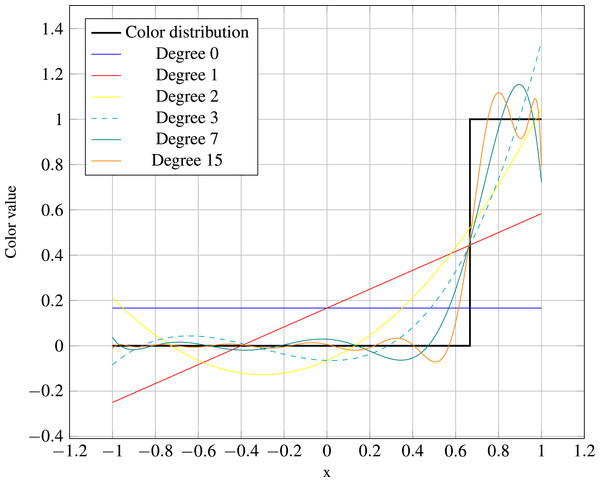Figure 2: Projection of a step function (6), jumping at x=23 onto the space of Legendre polynomials. Shown is the step function along with its approximation by more and more Legendre basis functions obtained by analytical integration.   Download full-size image DOI: 10.7717/peerj-cs.35/fig-2

However, before polynomials can be computed, the material distribution itself needs to be identified. Namely, we need to find regions of the domain where a specific material should be present. We achieve this by selectively attaching attributes to elements in the mesh. To define, which elements should be attributed and which not, we can exploit the flood filling algorithm explained above by enabling multiple fillings instead of just a single one. Individual surface descriptions confine each flooding, which allows the identification of distinct regions in the mesh. By ascribing a particular material definition to each of these regions, the voxelized spatial material distribution can be obtained. This approach is similar to coloring an image, and we refer to those floodings as colors. In the following, we briefly discuss the coloring concept and then move on to the generation of high-order surface representations within the Octree mesh.

### Coloring

Seeder takes a surface triangulation along with a seed definition to construct the computational domain with non-overlapping cubical elements. The seeds are usually points, but might also be other geometrical objects and are used as the starting point for the flood-filling algorithm. The surface description builds the confinement for the flood-filling. These two parts together are therefore building the volumetric geometry definition in the mesh. By using multiple of such pairs, it gets possible to describe different regions within the same mesh. We refer to this as coloring, and each seed and surface needs to have a color attached to it.

The flooding spreads from the seeds and is limited by surfaces of the same color. Boundaries of other colors do not affect the flooding and it is possible to have elements flooded by multiple colors. Differently colored regions might, therefore, overlap. The color information is then attached to the elements and provide a method to distinguish specific areas in the mesh. Afterward, the solver can associate individual material properties to given colors.

### Sub-resolution

With the coloring principle described above, we are now able to define arbitrary material areas, but we still need to obtain high-order surface approximations inside the elements of the Octree. As this provides information beyond the resolution of the actual mesh, we refer to this as sub-resolution. Figure 3 sketches the overall workflow of the algorithm to construct this information.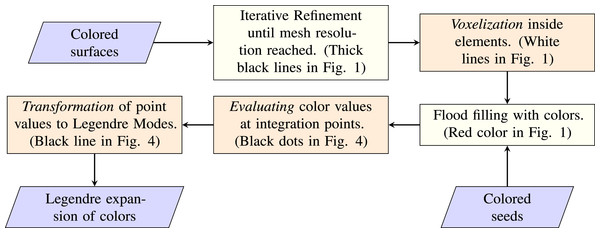Figure 3: Chart of the overall workflow. Required inputs are the surface descriptions and seeding points to start the flooding. The resulting output is the expansion of the color distribution in Legendre modes for each element.   Download full-size image DOI: 10.7717/peerj-cs.35/fig-3

To obtain the sub-resolution as an expansion in Legendre polynomials in each element, we need to perform the following three steps:

• Voxelization (and flooding) within elements of the final mesh to identify color distributions

• Evaluation of color values at integration points

• Transformation of point data to polynomial modes

These three steps are indicated by a darker orange color in Fig. 3. The other two processes are already described above. A first step creates the coarse mesh with the desired resolution, and after resolving the surfaces within the elements by the voxelization process, all elements and voxels are flood filled with colors.

The first additional step we introduce is the identification of color boundaries inside the coarse mesh elements. Luckily, we already have a robust and fast method to identify color boundaries in volumes by the voxelization method described above for the mesh. We can deploy this mechanism also inside elements and recursively refine the voxels towards surfaces. The final mesh will not contain the voxels within the coarse elements but rather the polynomial approximation of the color distribution. We limit the refinement inside elements by setting the number of additional levels ℓ. This number can be freely chosen in the configuration and all elements intersecting a boundary will be refined accordingly, independent of the size of the coarse element.

We observe that even though the voxelization is only a first order approximation, it is feasible to represent the surface accurately due to the exponential nature of the bisection approach in the Octree. After all voxels are known, the mesh generation algorithm proceeds with flooding as described in the previous section. The flood filling does not heed the intersected coarse elements of the final mesh. Instead, all voxels are flooded down to the finest level. With this approach, we do not require a separate algorithm for the flooding of voxels inside intersected elements.

Figure 1 illustrates the mesh status after refinement and flooding for eight coarse elements intersected by a sphere. The sphere is indicated by the yellow surface and cut open to reveal the voxelization within. Thick, black lines indicate the coarse mesh elements and the fine white lines show the sub-resolution voxels within them. As described above, elements in the interior of the sphere are flooded, which is indicated by the red coloring. The flooding is limited by the sphere and all voxels outside the geometry are not flooded with this color.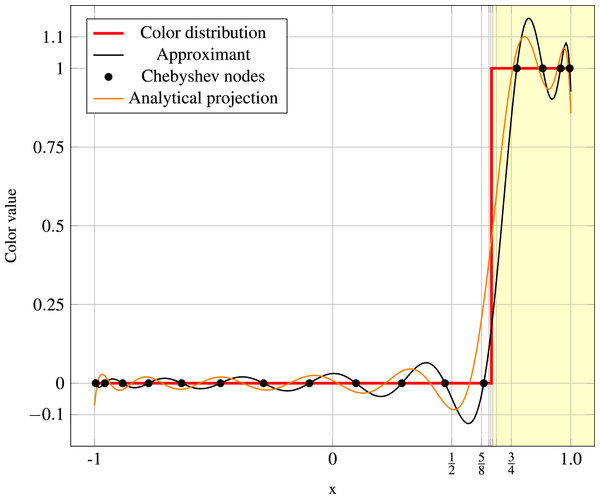Figure 4: Illustration of the approximation method in 1D. For a single element and one discontinuity. The color value jumps from 0 to 1 at x=23 and is indicated by the red line. The grid lines indicate the bisection sequence and the yellow area highlights the region, where the color value is identified to be 1 by the bisecting approximation. An approximant polynomial of degree 15 is constructed from the 16 shown Chebyshev nodes (black dots). The orange polynomial shows the analytical projection with degree 15, also depicted in Fig. 2.   Download full-size image DOI: 10.7717/peerj-cs.35/fig-4

Two processes remain to be done at this stage, the evaluation of color values and their transformation to Legendre modes. These two are hard to illustrate in three dimensions, and we will instead make use of the one-dimensional setup in Fig. 4. It makes use of the same target step function Ξ with $\xi =\frac{2}{3}$, as the one in Fig. 2, but outlines the individual numerical steps we take to arrive at an approximation of the analytical projection. Vertical grid lines indicate the recursive voxelization towards the surface point. We assume the flooding to happen right of the surface, that is, the seed is at some location x > 1. This flooding is indicated by the yellow shaded area in Fig. 4. Thus, the numerical method has to approximate a step function (6) with $\xi =\frac{2}{3}$, illustrated in the figure by the thick red line. With eight bisections in the voxelization, this results in an approximation of the jump location at $\stackrel{ˆ}{\xi }=0.671875$. This state corresponds to the three-dimensional case outlined in Fig. 1.

The number of additional refinement levels ℓ determines the spatial accuracy of the surface approximation. However, they only build one half of the numerical approximation; the other half is governed by the quality of the polynomial representation that we construct in the final two steps. The accuracy of our polynomial approximation is determined by the number of Chebyshev nodes N at which the color distribution is evaluated. For a given N, Chebyshev nodes are given by ${x}_{c}=cos\left(\frac{2c-1}{2N}\pi \right),\phantom{\rule{1em}{0ex}}c=1,\dots ,N.$ Both factors, ℓ and N, can be set independently by the user. However, they both limit the accuracy of the overall approximation, and for optimal results, they need to be correlated. The minimal distance between the first Chebyshev node and the element boundary is proportional to N−2, and the length of the smallest voxel within the element is proportional to 2−ℓ. To resolve all node distances, it is, therefore, necessary to choose the number of additional levels ℓ according to $\ell \ge ⌈2{log}_{2}\left(N\right)⌉.$

Once the flooding situation of all voxels is known, we can evaluate the color at each Chebyshev node. For this, numerical values need to be associated with the flooding status for each color. Usually, we assume a value of 1 for flooded voxels and a value of 0 for non-flooded voxels. Due to the spatial discretization by an Octree, the color value at each Chebyshev node xc can be found fast with logarithmic computational complexity. In Fig. 4 the Chebyshev nodes (8) for N = 16 are indicated by black dots and their color value by the elevation of the dots. The approximated step function provides the color values, and we obtain for the polynomial values at the Chebyshev nodes xc. It is notable that the position of the surface is only significant up to the interval between two neighboring nodes. A variation of the jump location within the interval between two neighboring nodes does not change the final approximation. The only option to increase the accuracy of the approximation is to use a larger number of integration points N.

Finally, the transformation of the nodal information (10) to a suitable function space for the solver has to be done. A typical choice for discontinuous Galerkin methods is the orthogonal Legendre basis. To obtain the Legendre modes ai in (7) from the values at the Chebyshev nodes fc in (10), we apply the fast polynomial transformation proposed in Alpert & Rokhlin (1991). However, other target functions with different point sets could also be plugged into the described machinery. The obtained polynomial recovers the function values fc at the nodes exactly and is drawn in Fig. 4 by the black line running through all dots. Due to the discontinuity of the step function, the representation in polynomial space is an infinite series. The finite approximation suffers from the Gibbs phenomenon (Wilbraham, 1848). However, besides this fundamental problem, also inaccuracies due to the numerical integration can be seen as the numerical (black) and the analytical (orange) projections do not coincide in Fig. 4. These result in a distorted location of the jump. In the next section, we will have a closer look at these numerical issues.

With this step, we now have a volumetric description that yields a high-order approximation of the surface. Note that only intersected coarse mesh elements need to get this information added, all other elements have constant colors. Thus, the need for volume information is limited to a small area at the surface.

## Numerical Properties

In this section, we investigate the numerical properties of the described approximation method. Though, the one-dimensional problem with a single jump is much simpler than a real three-dimensional geometry; it is still instructive for the fundamental properties of the algorithm. Let us recall that the goal is an appropriate representation of the geometry in a high-order discontinuous Galerkin solver. Typically, the deployed functions in the solver are smooth within elements. Here we consider specifically Legendre polynomials, which are attractive due to their orthogonality. The representation of a non-smooth material distribution in the finite smooth function space, therefore, can only be approximate.

Table 1 shows the convergence behavior for the series of Legendre polynomials, obtained by L2 projections of the step function at $x=\frac{2}{3}$ in the interval [ − 1, 1]. A slow convergence can be observed, which is well known for high-order approximations of discontinuous functions. However, the error outside a small band around the discontinuity can be improved later on by a post-processing step, as shown in Gottlieb & Hesthaven (2001). The error is bound to this band around the discontinuity, and the width of that band decreases with the number of degrees of freedom. While we can compute this analytic projection for the simple setup with a single discontinuity in one dimension, this not possible anymore for multiple dimensions and more complex geometries. Thus, we need the previously described numerical approach to approximate the projection. In the following, we first analyze how well the numerical scheme recovers the optimal solution given by the L2 projection for the simple one-dimensional discontinuity.

Figure 5 shows the convergence of the numerical procedure towards the analytical projection onto a polynomial space with a maximal degree of 15. Plotted is the error over the spatial resolution, where the spatial resolution is given by the number of Chebyshev nodes used for the numerical integration. The difference in terms of the L2 norm to the analytical projection is represented by the blue line and covers all modes of the polynomial. With the red line, the absolute difference in the volume is provided. Note that the volume equals to the first mode of the Legendre polynomial and thus, is easily obtained. Nevertheless, the two curves show a similar behavior, and the volume error can be used as a good indicator of the qualitative behavior of this numerical approximation. Apparently, the error does not converge uniformly, but on average we observe a convergence rate of roughly 1. This error is comparable to the one due to the voxelization, and so these two resolutions (number of voxels and number of integration points) should be in the same range.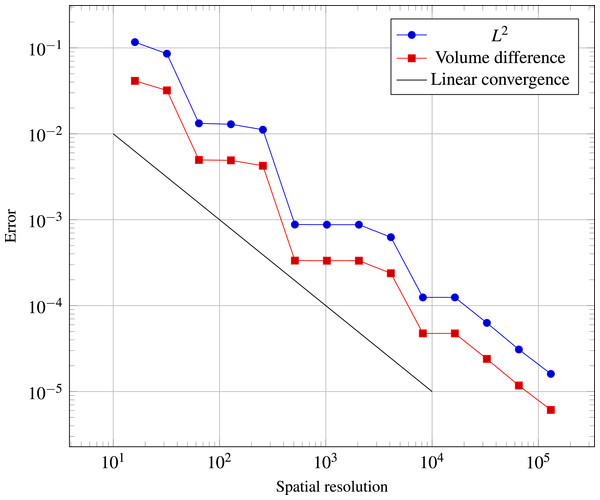Figure 5: Error convergence of the numerical approximation towards the analytical projection for a polynomial of degree 15. The blue line shows the L2-error over all 16 modes while the red line shows the absolute error in the first mode, which represents the volume. On average, a convergence rate of 0.973 is achieved. Keep in mind that in comparison to the actual step function, the error from Table 1 always remains.   Download full-size image DOI: 10.7717/peerj-cs.35/fig-5

To investigate the mechanism in 3D, we look at three different geometrical objects: a sphere, a cube, and a tetrahedron. In each case the overall domain is a cubical box [ − 1, 1] × [ − 1, 1] × [ − 1, 1] subdivided by 8 cubical elements. The sphere is put in the middle of the domain, with its center at (0, 0, 0) and has a radius of $\frac{1}{3}$. Similarly, the cube has an edge length of $\frac{2}{3}$, and its barycenter is placed at the center of the domain at (0, 0, 0). As a third basic geometry, the tetrahedron again is similarly defined with its barycenter in the center of the domain and an edge length of 1.

The error in the volume approximation is used to assess the quality of the polynomial representation. Figure 6 plots this measure for the sphere over the two available parameters voxelization resolution and numerical integration points. It can be observed that the error is mostly bounded by either one of the parameters and for a minimal computational effort it indeed is necessary to change them according to the relation (9).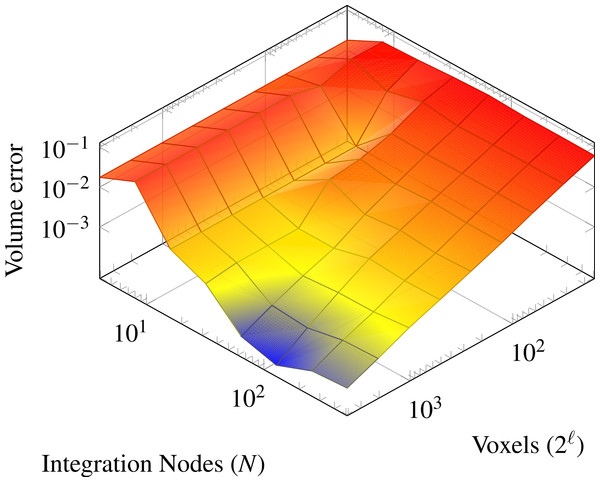Figure 6: Error in the volume approximation for a sphere with a radius of 13. The mesh consists of 8 elements with a common vertex in the center of the sphere.   Download full-size image DOI: 10.7717/peerj-cs.35/fig-6

Figure 7 illustrates the projection of the sphere on a 3D polynomial representation in the eight elements of the mesh. From 7A to 7D it shows improved accuracies. In yellow the isosurface of a color value of 0.5 is shown and in comparison the half of the reference sphere is shown in blue. Figure 7A shows the sphere embedded in the eight elements, indicated by the black wireframe. In this, a very rough estimation of the sphere is shown with a polynomial of degree 15 and a low voxelization resolution. Clearly, the staircases from the voxelization are visible in the polynomial representation here. The next image in 7B shows a zoom in for finer voxelization, but still a polynomial degree of 15, in the left half the reference sphere is again depicted in blue. While the finer resolution in the voxelization yields a better approximation now, there are relatively strong oscillations visible, especially close to the element boundaries. To allow for a better representation of the sphere we move to a polynomial degree of 31 in Fig. 7C. The voxelization is chosen with an appropriate resolution, in this case, but the numerical integration results in aliasing issues, exhibiting a staircase-like effect for the isosurface of the polynomial. Finally, in the Fig. 7D, we see the impact of a higher number of points for the numerical integration, resulting in a much smoother geometry for the shown polynomial of degree 31.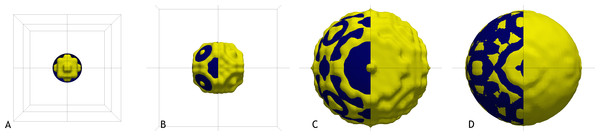Figure 7: Illustration of sphere approximations, with increasing accuracy. The sphere is blue, and the isosurface of the color value at 0.5 is yellow. In (A), the sphere is shown in the embedding domain with the 8 elements. Voxelization and integration points increase from (A) to (D).   Download full-size image DOI: 10.7717/peerj-cs.35/fig-7

Figure 8 illustrates the approximation of a cube. All images show the isosurface for polynomial representation of degree 15, but the accuracy of the numerical approximation increases from left to right. The number of integration points increases from 16 in Fig. 8A over 32 and 48 to 64 in Fig. 8D and the voxelization is chosen according to Eq. (9). Edges and corners get smoothed out, but we observe only little oscillations for this simple, axis aligned, geometry.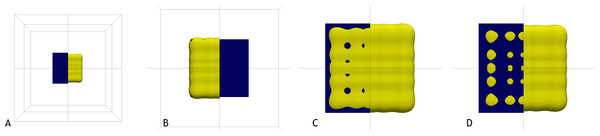Figure 8: Representation of the cube in 8 elements with polynomials of degree 15. From (A) to (D) an increasing number of integration points is used. Image (D) shows the cube with the 8 elements of the mesh. The reference geometry is drawn in blue, and the isosurface of the color value 0.5 in yellow. We cut the reference in the middle to enable a better view for the comparison, except for image (B) where it is the other way around, and the isosurface is cut.   Download full-size image DOI: 10.7717/peerj-cs.35/fig-8

Finally, Fig. 9 depicts a study on the 3D polynomial approximation of a tetrahedron. The maximal polynomial degree increases from 7 up to 63, and twice as many integration points as polynomial modes are used in each approximation. We observe that the sharp edges and corners are smoothed out at low orders but are increasingly well recovered in the higher resolved polynomials. Also, oscillations in the planes of the tetrahedron get smaller in amplitude. With a polynomial of degree 63, the original shape is well captured. This shows that a high-order polynomial can nicely be constructed, even for objects with sharp corners and edges.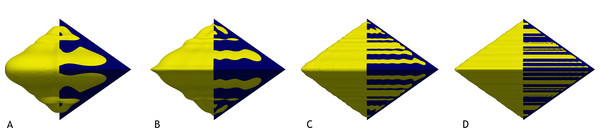Figure 9: Approximation of the tetrahedron with an increasing polynomial degree from (A) to (D). Starting in (A) with a polynomial degree of 7 and increasing over 15 and 31 to 63 in image (D). Shown is the isosurface of the polynomial at a value of 0.5 in yellow and for comparison the reference geometry cut in half with a blue coloring.   Download full-size image DOI: 10.7717/peerj-cs.35/fig-9

Figure 10 shows the application of the described method to a more complex geometry. Depicted is in yellow the isosurface of a polynomial approximating a porous medium, described by a triangulation in an STL file shown in dark blue. This geometry features small bridges and holes. Those are well recovered by the polynomial approximation; only the edges get a little bit smoothed out. Keep in mind, that we are only using cubical elements, which can be exploited by the numerical scheme. Also, no bad elements arise resulting in an extremely robust mesh generation.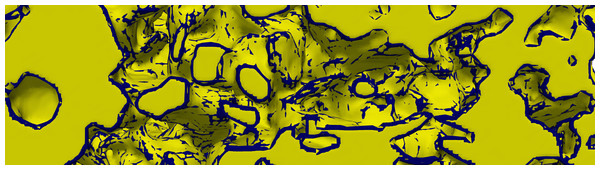Figure 10: Isosurface of a porous medium (yellow) in comparison to the original STL data (blue). The geometry is well recovered; only edges are smoothed out a little.   Download full-size image DOI: 10.7717/peerj-cs.35/fig-10

## Application in DGFEM for Electrodynamics

To show the behavior of the obtained geometry, we look at an electrodynamic setting, governed by Eqs. (1)(4), and solve it with a high-order, modal DGFEM. For time integration, a classical explicit fourth order Runge–Kutta integration is deployed. The simulation setup is an infinite cylinder, impinged by a planar wave. In this setting, the scattering of the wave can be described by a Mie series (Mie, 1908), which we use as the reference solution. Our simulation setup has the following parameters:

• Permittivity and permeability in the surrounding: εS = μS = 1

• Permittivity and permeability in the cylinder: εC = μC = 2

• Simulated domain: [ − 1, 1] × [ − 1, 1] × [0, 0.125]

• Radius of the cylinder r = 0.21

• Center of the cylinder: (0.0009765625, 0.0009765625)

• The impinging planar wave has a wave length of λ = 0.25

The domain is discretized with 16 × 16 × 1 = 256 elements, and polynomials with a maximal polynomial degree of 15 are used to represent the solution in each element. For the initial condition of the numerical simulation, we also employ the Mie series. We compare the numerical result with the reference solution after the impinging wave has traveled once by the diameter of the cylinder.

In Fig. 11A the instanteneous exact solution for the Z-component of the displacement field D = εE is shown. Right to this reference solution, the difference between the numerical solution and this reference after a simulation time of 0.42 is depicted in Fig. 11B. We use a range in ±10% of the maximal amplitude in the exact solution for the scale of the difference. A de-aliasing is applied in the numerical scheme here. Thus, while the scheme uses 16 modes per direction to represent the solution, we use twice as many modes (32) to compute the multiplication of the material distribution with the solution. In the numerical simulation, no voxelization is employed. Instead, the exact definition of the cylinder is used to determine the material values at the 32 Chebyshev nodes per direction that are used to construct the polynomials with a maximal polynomial degree of 31. This corresponds to ℓ = ∞ with aa = 1 in Table 2. As can be seen, the reference solution is recovered quite accurately in the largest part of the domain. Only close to the actual interface, there are larger deviations observed. Please note that no smoothening post-processing was applied here, and all Gibbs oscillations are visible in the deviations.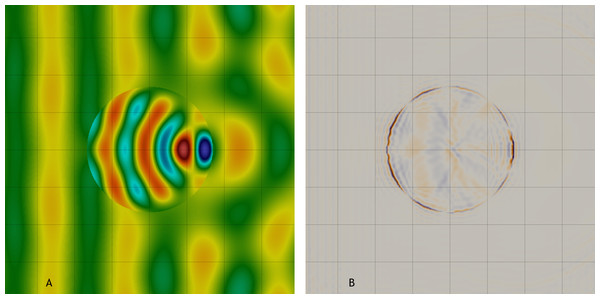Figure 11: Scattering of a planar wave at a cylindrical object. The grid lines indicate the mesh of the DGFEM solver. For the numerical solution, a basis with a maximal polynomial degree of 15 is used. In (A), the reference solution is shown. In (B), the difference between the numerical solution and the reference can be seen for a de-aliasing by 32 points. The color scale for the difference is chosen with a range of ±10% of the maximal amplitude in the reference.   Download full-size image DOI: 10.7717/peerj-cs.35/fig-11

We now replace the exact definition of the cylindrical geometry by the polynomial representation obtained by the method described above. All other parameters of the numerical setup remain the same. For all simulations, the cylinder geometry is approximated by polynomials with a maximal polynomial degree of 31 in each element. Similarly to Fig. 6, we can vary the number of integration points and the size of the smallest voxels in each element for the construction of these polynomials.

To judge the quality of the thereby obtained cylinder approximations for the DGFEM scheme, we build the L2-error across the 8 × 8 elements enclosing the cylinder. For the comparison, we consider the instantaneous solution after simulating a time interval of 0.42 (the time it takes the impinging wave to move once by the diameter of the cylinder). The errors are measured in the Z component of the displacement field D and are shown in Table 2. We increase the voxel resolution (ℓ) from row to row in Table 2. In the columns we increase the anti-aliasing, that is the number of Chebyshev nodes to construct the polynomials of degree 31. The aa factor is to be understood as a multiplicator, such that with aa = 1 we use 32 points per direction to construct the polynomials and with aa = 4 we use 128.

As can be seen in Table 2, the error always improves with the voxel resolution ℓ. A higher anti-aliasing, however, does not always improve the solution quality. This behavior matches the observations in Fig. 6 and emphasizes the necessity for voxel resolutions that resolve the smallest distances between Chebyshev nodes.

## Summary and Outlook

Seeder is a mesh generating tool providing an Octree representation with cubical elements to a solver. We enhanced these cubical elements in this work by polynomial representations of material distributions within each element. To obtain these polynomials, we employ a first order voxelization utilizing the Octree refinement strategy with a colored flood filling to identify the material distribution. Numerical integration then transforms those detailed material distributions into multi-dimensional Legendre polynomials. This approach is highly robust and applicable to complex geometries. It does not impose strong requirements on the quality of the surface description.

We have demonstrated that the proposed method indeed converges towards the optimal L2 projection of the nonsmooth target function onto the polynomial function space. The obtained geometry representation is suitable for high-order DGFEM solvers as we have shown in a small analysis for the scattering of a planar electromagnetic wave at a cylindrical obstacle.

A possible improvement to the current staircase representation of the surface could be achieved by computing an approximate plane for the geometry within intersected voxels. While such a computation would introduce additional complexity and potentially expensive computations, it would reduce the number of voxels required to resolve the shortest distances between integration nodes. Nevertheless, the simple voxelization scheme currently deployed is already capable of discretizing large and complex settings and has been successfully used for highly detailed simulations.

A general argument against high-order methods for scenarios with nonsmooth solutions is the bad convergence as given in Table 1. However, this can be overcome by an appropriate post-processing. In Zudrop & Hesthaven (2015) it is proven that high-order information can be recovered from solutions suffering from Gibbs oscillations. Such a post-processing step only needs to be applied to the final simulation result and has not been covered here. We believe the described geometry generation method enlarges the applicability of high-order methods to many settings with non-trivial geometries.

## Additional Information and Declarations

### Competing Interests

The authors declare there are no competing interests.

### Author Contributions

Harald G. Klimach wrote the paper, prepared figures and tables, performed the computation work, reviewed drafts of the paper.

Jens Zudrop performed the computation work, reviewed drafts of the paper.

Sabine P. Roller wrote the paper, reviewed drafts of the paper.

### Data Availability

The following information was supplied regarding data availability:

Bitbucket: https://bitbucket.org/apesteam/seeder.

### Funding

The authors received no funding for this work.